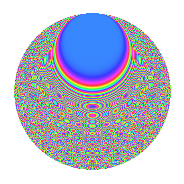# Properties

 Label 3150.2.cbLevel 3150 Weight 2 Character orbit cb Rep. character $$\chi_{3150}(443,\cdot)$$ Character field $$\Q(\zeta_{12})$$ Dimension 576 Sturm bound 1440

# Related objects

## Defining parameters

 Level: $$N$$ = $$3150 = 2 \cdot 3^{2} \cdot 5^{2} \cdot 7$$ Weight: $$k$$ = $$2$$ Character orbit: $$[\chi]$$ = 3150.cb (of order $$12$$ and degree $$4$$) Character conductor: $$\operatorname{cond}(\chi)$$ = $$315$$ Character field: $$\Q(\zeta_{12})$$ Sturm bound: $$1440$$

## Dimensions

The following table gives the dimensions of various subspaces of $$M_{2}(3150, [\chi])$$.

Total New Old
Modular forms 2976 576 2400
Cusp forms 2784 576 2208
Eisenstein series 192 0 192

## Trace form

 $$576q$$ $$\mathstrut -\mathstrut 16q^{6}$$ $$\mathstrut +\mathstrut O(q^{10})$$ $$576q$$ $$\mathstrut -\mathstrut 16q^{6}$$ $$\mathstrut +\mathstrut 288q^{16}$$ $$\mathstrut -\mathstrut 36q^{17}$$ $$\mathstrut -\mathstrut 16q^{18}$$ $$\mathstrut +\mathstrut 24q^{21}$$ $$\mathstrut -\mathstrut 36q^{27}$$ $$\mathstrut +\mathstrut 20q^{33}$$ $$\mathstrut -\mathstrut 24q^{41}$$ $$\mathstrut +\mathstrut 4q^{42}$$ $$\mathstrut +\mathstrut 24q^{46}$$ $$\mathstrut +\mathstrut 48q^{51}$$ $$\mathstrut +\mathstrut 48q^{57}$$ $$\mathstrut -\mathstrut 24q^{58}$$ $$\mathstrut -\mathstrut 24q^{61}$$ $$\mathstrut +\mathstrut 28q^{63}$$ $$\mathstrut -\mathstrut 8q^{72}$$ $$\mathstrut +\mathstrut 48q^{77}$$ $$\mathstrut +\mathstrut 16q^{78}$$ $$\mathstrut -\mathstrut 8q^{81}$$ $$\mathstrut -\mathstrut 40q^{87}$$ $$\mathstrut +\mathstrut 24q^{92}$$ $$\mathstrut +\mathstrut 12q^{93}$$ $$\mathstrut -\mathstrut 8q^{96}$$ $$\mathstrut +\mathstrut O(q^{100})$$

## Decomposition of $$S_{2}^{\mathrm{new}}(3150, [\chi])$$ into irreducible Hecke orbits

The newforms in this space have not yet been added to the LMFDB.

## Decomposition of $$S_{2}^{\mathrm{old}}(3150, [\chi])$$ into lower level spaces

$$S_{2}^{\mathrm{old}}(3150, [\chi]) \cong$$ $$S_{2}^{\mathrm{new}}(315, [\chi])$$$$^{\oplus 4}$$$$\oplus$$$$S_{2}^{\mathrm{new}}(630, [\chi])$$$$^{\oplus 2}$$$$\oplus$$$$S_{2}^{\mathrm{new}}(1575, [\chi])$$$$^{\oplus 2}$$